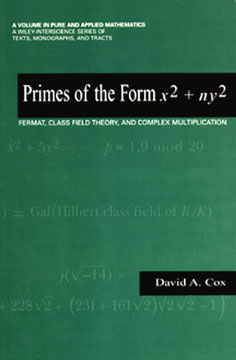# ZZ Top and the Heros of MathematicsMy summer reading,$textrm{Primes of the form}, x^2 + ny^2"$, follows the mathematical discoveries and subsequent works of Joseph Lagrange, Leonhard Euler, Adrien Legendre, and Carl Gauss. My goal in mathematics is to make the kinds of significant discoveries that these mathematicians made.

Euler lived in the 18th century; he proved some theorems about primes of the form$x^2 + ny^2$ which had been conjectured in the 17th century by another famous mathematician, Pierre de Fermat. Lagrange, who succeeded Euler as the director of the Prussian Academy of Sciences, and Legendre both studied quadratic forms and their applications to Euler’s work. One of the applications of the theory of quadratic forms is to generalize Euler’s proofs. However, Legendre developed the theory in a much more modern and natural way. In addition, he made significant developments in the theory of quadratic reciprocity. Finally, Gauss synthesized all of the above ideas. He is widely considered to be the finest mathematician ever.

I am fascinated by who these famous mathematicians were, whereas during my engineering studies I was more interested in the how and why questions. For example, there is a famous result in algebraic number theory credited to Ernst Kummer: the number field generated by the 23rd root of unity does not have unique factorization while the number fields generated by the nth root of unity for$n < 23$, had all been found to have unique factorization. My question was, “how the heck was Kummer able to figure this out?” to which my professor responded, “…he was some kind of genius.”

I may not be a genius in the same class as Kummer and Gauss, but I wouldn’t be studying mathematics if I didn’t enjoy it or if I didn’t think I could be successful in it. I hope one day, I will be in a position to make a significant mathematical discovery on par with the heroes of mathematics.

This entry was posted in General. Bookmark the permalink.